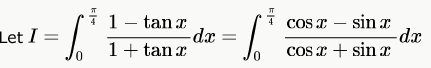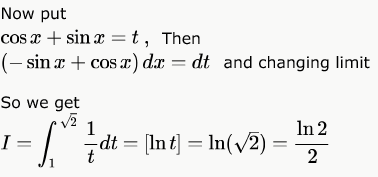Courses

# Calculus MCQ - 1

## 20 Questions MCQ Test RRB JE for Computer Science Engineering | Calculus MCQ - 1

Description
This mock test of Calculus MCQ - 1 for Computer Science Engineering (CSE) helps you for every Computer Science Engineering (CSE) entrance exam. This contains 20 Multiple Choice Questions for Computer Science Engineering (CSE) Calculus MCQ - 1 (mcq) to study with solutions a complete question bank. The solved questions answers in this Calculus MCQ - 1 quiz give you a good mix of easy questions and tough questions. Computer Science Engineering (CSE) students definitely take this Calculus MCQ - 1 exercise for a better result in the exam. You can find other Calculus MCQ - 1 extra questions, long questions & short questions for Computer Science Engineering (CSE) on EduRev as well by searching above.
QUESTION: 1

### Let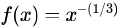and  denote the area of region bounded by f(x) and the X-axis, when  varies from -1 to 1. Which of the following statements is/are TRUE? I.  is continuous in [-1, 1] II.  is not bounded in [-1, 1] III.  is nonzero and finite

Solution:

I. False.
II. True.
III. True. An area is always positive, while the definite integral might be composed of several regions, some positive and some negative. A definite integral gets you the net area, because any part of the graph that is below the x-axis will give you a negative area. So, a definite integral is not necessarily the area under the curve, but the value of the area above the x-axis less the area under the x-axis. So, A is non-zero and finite.

QUESTION: 2

### The function y=|2 - 3x|

Solution: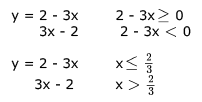as y is polynomial it is continuous and differentiable at all points but don't know at = 2/3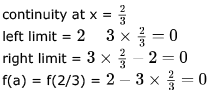LL = RL = f(a) so y is continuous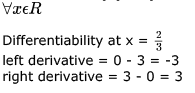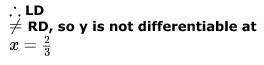QUESTION: 3

### Which one of the following functions is continuous at x = 3?

Solution:

For continuity, Left hand limit must be equal to right hand limit. For continuity at x = 3, the value of f(x) just above and just below 3 must be the same.

A. f(3) = 2. f(3+) = x - 1 = 2. f(3-) = (x+3)/3 = 6/3 = 2. Hence continuous.
B. f(3) = 4. f(3+) = f(3-) = 8 - 3 = 5. So, not continuous.
C. f(3) = f(3-) = x + 3 = 6. f(3+) = x - 4 = -1. So, not continuous.
D. f(3) is not existing. So, not continuous.

QUESTION: 4

A function f(x) is continuous in the interval [0,2]  f(0) = f(2) = -1 and f(1) = 1.  Which one of the following statements must be true?

Solution:

Let's define a new function g,
g(y) = f(y) -f(y+1)
Since function f is continuous in [0,2], therefore g would be continuous in [0,1] g(0) = -2, g(1) = 2
since g is continuous and goes from negative to positive value in [0,1]. therefore at some point g would be 0 in (0,1).
g=0 ⇒ f(y) = f(y+1) for some y in (0,1)

Therefore, correct answer would be (A).

QUESTION: 5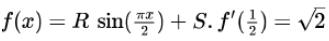and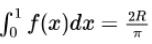, then the constants  and  are

Solution: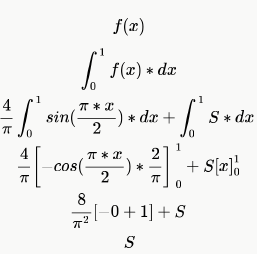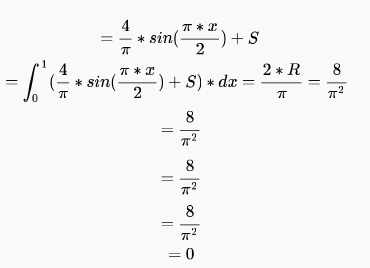*Answer can only contain numeric values
QUESTION: 6

Let f(x) be a polynomial and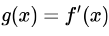be its derivative. If the degree of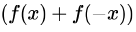is 10, then the degree of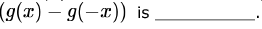Solution: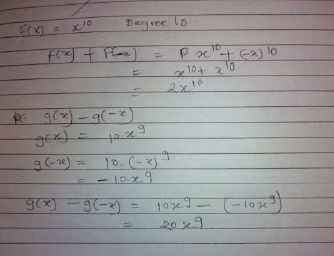F is some function where the largest even degree term is having degree  10. no restiction on odd degree terms.
since f(x)+f(-x)= degree 10
even power gets converted to odd in derivative.
then the the degrre of required expression =9.
the odd powers in F will become even in derivative and G(X)-G(-X) retains only odd powers.

QUESTION: 7

Consider the following two statements about the function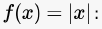• P. f(x) is continuous for all real values of .
• Q .f(x) is differentiable for all real values of  .

Which of the following is TRUE?

Solution:

f(x)=|x| here for all values of x, f(x) exists. therefore it is continuous for all real values of x. At x=0, f(x) is not differentiable. Because if we take the left hand limit here, it is negative while the right hand limit is positive.

QUESTION: 8

Consider the function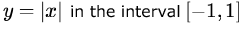. In this interval, the function is

Solution:

It is continuous but not differentiable at x=0 as left hand limit will be negative while the right hand limit will be positive but for differentiation, both must be same.

*Answer can only contain numeric values
QUESTION: 9

Let f be a function defined by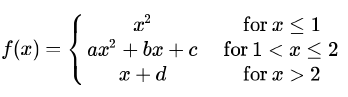Find the values for the constants a, b,c  and d so that f is continuous and differentiable everywhere on the real line.

Solution: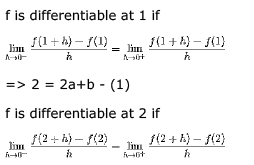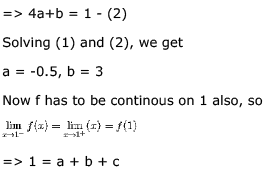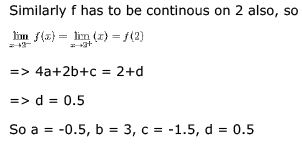QUESTION: 10

Let the function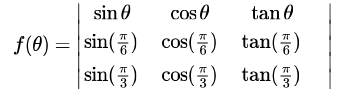where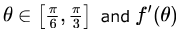denote the derivative of f with respect to θ. Which of the following statements is/are TRUE?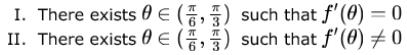Solution:

We need to solve this by rolle's theorem, to apply rolle's theorem following 3 conditions should be satisfied:
1) f(x) should be continuous in interval [a, b],
2) f(x) should be differentiable in interval (a, b), and 3) f(a) = f(b)
If these 3 conditions are satisfied simultaneously then, there exists at least one 'x' such that f '(x) = 0
So, for the above question, it satisfies all the three conditions, so we can apply rolle's theorem, i.e, there exists 'at least one' theta that gives f '(theta) = 0
Also, the given function is also not a constant function, i.e f '(theta) ≠ 0

*Answer can only contain numeric values
QUESTION: 11

The function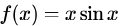satisfies the following equation: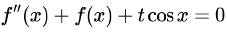. The value of  is______.

Solution: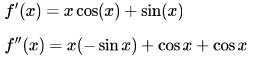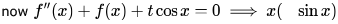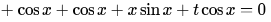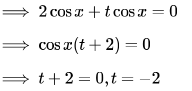QUESTION: 12

The formula used to compute an approximation for the second derivative of a function f at a point x0 is

Solution:
QUESTION: 13

In the interval  [0,π] the equation x = cos x has

Solution:

f you consider x=0 then cosx=1
now if x= PI/4 = 0.785 then cosx=0.7071
for some x value x=cosx
after this x is increasing and cosx is decreasing. so we can say exactly 1 solution.

EDIT-
It is very easy to show that the equation x = cos x has a unique solution. For example take f(x) = c - cos x and notice that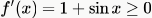(equality holding in isolated points) so f(x) is strictly increasing and hence the equation can have at most one solution.

At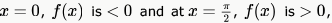and function is continious (difference of two continuous functions is continuous). Therefore there is solution in ,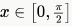hence there is a solution in [0,π]

QUESTION: 14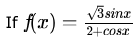then the range of f(x) is

Solution: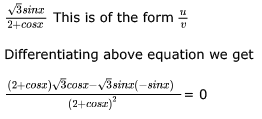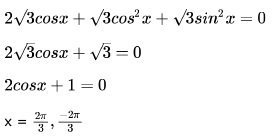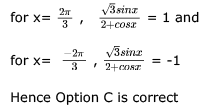QUESTION: 15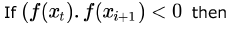Solution:

as f(xi).f(xi+1) <0
means one of them is positive and one of them in negative . as their multiplication is negative
so when you draw the graph for f(x) where xi<=x<=xi+1. Definitely F(x) will cut the X- axis.
so there will definitely a root of F(x) between xi and xi+1

QUESTION: 16

If  f(x) = x2 and g(x) = x sinx +cosx then

Solution: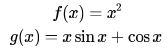We are asked to make this two function equal and see at how many points they meet.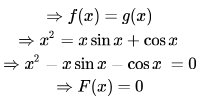This F(x) is even and we just need to check at how many points F(x) crosses the X - axis for x > 0.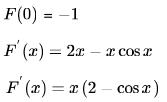Here 2-cos x is greater than 0 and  (2 - cosx) will be also greater than  for x > 0.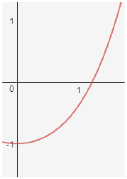As we have already seen that F(x) is even, therefore F(x) has only two real roots.

QUESTION: 17

Given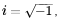what will be the evaluation of the definite integral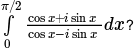Solution: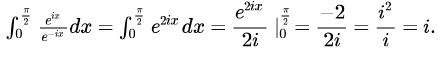QUESTION: 18

What is the value of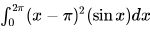Solution:

Put  x - π = t then limit 0 changes to  - π and upper limit 2π changes to π.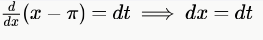Integration of  t2 for limit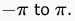to . One is an odd function and one is even and product of odd and even functions is odd function and integrating an odd function from the same negative value to positive value gives 0.

QUESTION: 19

If for non-zero x,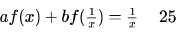where a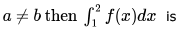Solution: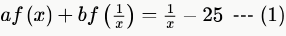Integrating both sides,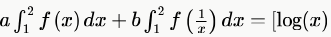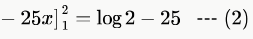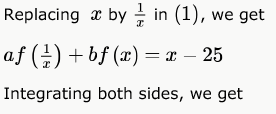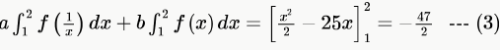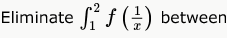(2) and (3) by multiplying (2) by  and (3) by  and subtracting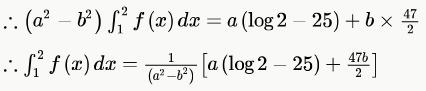QUESTION: 20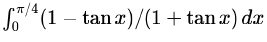Solution: